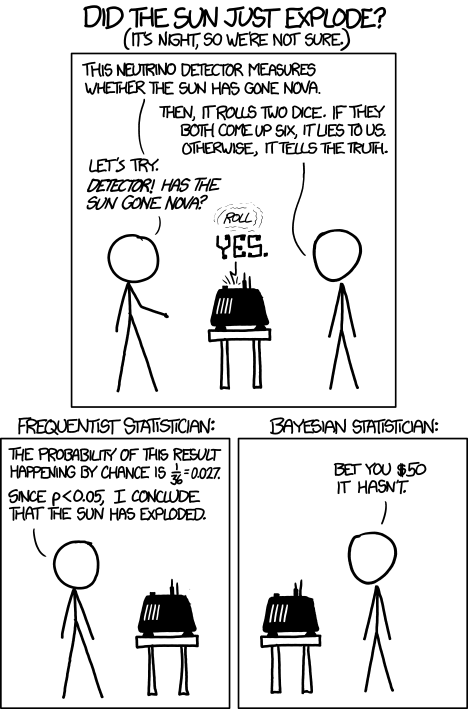## Thursday, March 31, 2016

### Eyewitness Testimony and Miracles

I mentioned in my review of the William Lane Craig vs. Alex Rosenberg debate that the “eyewitness testimony is unreliable” objection is a bad one to use against e.g. arguments for the resurrection of Jesus. Here’s a somewhat obvious problem if eyewitness testimony is unreliable to the point where anything relying on eyewitness testimony should not be accepted as true: if you follow that rule consistently, then pretty much every fact of ancient history is tossed in the rubbish bin.

At the very least the “eyewitness testimony is unreliable” objection needs to be fine-tuned more; preferably there should be some explanation for why we shouldn’t trust eyewitness testimony in the case of miracles even though eyewitness testimony is often reliable in many other cases (remember, pretty much every scientific fact we have relies on eyewitnesses who recorded e.g. results of experiments; think of that next time you read a physics textbook). That’s what I’ll do in this article.

Happily, there’s an XKCD comic to help illustrate the idea. Behold:In this case the neutrino detector serves as a representation for a generally reliable eyewitness (it’s accurate about 97.2% of the time), and the sun explosion represents a fantastical event with an extremely low prior probability. Don’t know what a prior probability is? Here’s one version of Bayes’ theorem:

 P(H|E) = P(H) × P(E|H) P(E)

In this case, P(H) represents the prior probability. Let’s use the following symbolization key:

• H = The sun exploded
• ¬H = The sun did not explode.
• E = The neutrino detector reports the sun has exploded.

Suppose there’s a one in a million shot that the sun exploded (I’m pretty sure that’s an overestimate, but this will suffice for our purposes).

 P(H) = 1 1,000,000

The probability that our neutron detector is telling us a falsehood is 1 in 36. So on average, of the times that the sun does not explode, it will inaccurately report it 1/36 of the time. Thus:

 P(E&¬H) = 1 × 999,999 ≈ 0.027778 36 1,000,000

Of the times that the sun does explode, it correctly reports it 35/36.

 P(E&H) = 35 × 1 ≈ 0.00000097222 36 1,000,000

Note that since the sun not exploding is a far more likely event, the frequency of false positives (≈ 2.778% of the time) vastly outweighs the frequency of true positives (≈ 0.000097222% of the time) given that the neutrino detector reports the truth only 35/36 of the time. According to the law of total probability:

P(E) = P(E&H) + P(E&¬H) ≈ 0.027778

Also, given the following equation and plugging in the values above for P(E&H) and P(H):

 P(E|H) = P(E&H) ≈ 0.97222 P(H)

Plugging in our values for Bayes’ theorem gives us this:

 P(H|E) = P(H) × P(E|H) ≈ 0.000035 P(E)

In the XKCD comic, the “Frequentist Statistician” committed what’s called the base rate fallacy, concluding that the sun probably exploded because the “base rate” reliability of the neutrino detector seems (to this statistician) to suggest the sun probably exploded. The problem, as I suggested before, is that on average the frequency of false positives vastly outweighs the frequency of true positives. This sort of effect is known as the paradox of the false positive, a.k.a. the false positive paradox. Very roughly, this paradox is where false positives are more likely than true positives when the overall high reliability of the “test” (most of the times the test results accurately report the situation) might lead one to believe that a positive result (e.g. the detector saying the sun exploded) means that a positive case is likely true.

What’s our application to eyewitness testimony and miracles? Miracles have extremely low prior probabilities, and as the case of the XKCD comic illustrates, with a low enough prior probability a fairly reliable information source could lead to false positives being more likely than true positives. This sort of thing is why we can rationally reject crazy alleged eyewitness reports like being abducted by aliens. It is also why we can be skeptical of alleged eyewitness testimony of miracles.